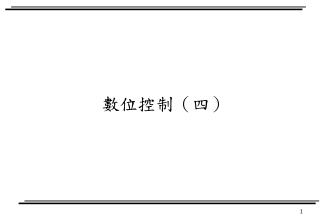# 數位控制（四） - PowerPoint PPT PresentationDownload PresentationDownload Presentation## 數位控制（四）

- - - - - - - - - - - - - - - - - - - - - - - - - - - E N D - - - - - - - - - - - - - - - - - - - - - - - - - - -
##### Presentation Transcript

1. 數位控制（四）

2. Polynomial in z or z-1 It is preferable to express X(z) as a ratio of polynomials in z, rather than z-1.

3. Inverse z transform • Direct division method • Computational method • MATLAB approach • Difference equation approach • Partial-fraction-expansion method • Inversion integral method

4. Direct division method

5. MATLAB approach X(z) G(z) Y(z) invz.m x(k) y(k) G(z)

6. Difference equation approach

7. Partial-Fraction-Expansion Method

8. Partial-Fraction-Expansion Method

9. Inversion Integral Method

10. Z transform method for solving difference equation

11. Difference equation example

12. z Plane Analysis of Discrete-Time Control System • z transform method is useful for Single-Input-Single-Outout (SISO) system. • Multi-Input-Multi-Outout (MIMO) will be introduced in Chapter 5. • It enable us to apply conventional continuous-time design method to discrete-time system. • s domain technique

13. SISO / MIMO

14. Impulse sampling

15. Exercise 2 • Ogata • B-2-8 • B-2-9